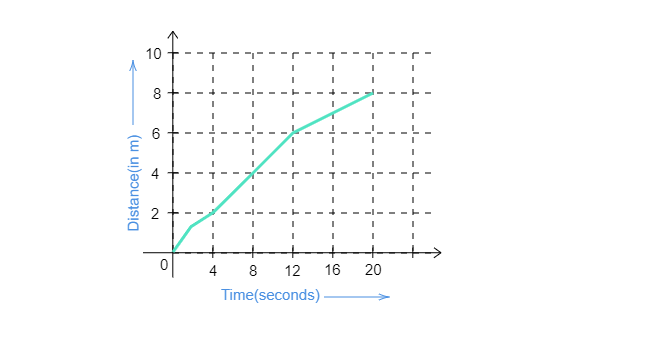# Figure given below is the distance-time graph of the motion of an object.$(i)$. What will be the position of the object at 20 s?$(ii)$. What will be the distance traveled by the object in 12 s?$(iii)$. What is the average speed of the object?"

$(i)$. At $20\ s$, the object will be $8\ m$ away.

$(ii)$. From the graph, it is clear, that in $12\ s$ the object will travel $6\ m$.

$(iii)$. From the given graph, total distance $=8\ m$

Total time $=20\ s$

Average speed $=\frac{distance}{time}$

$=\frac{8\ m}{20\ s}$

$=0.4\ m/s$